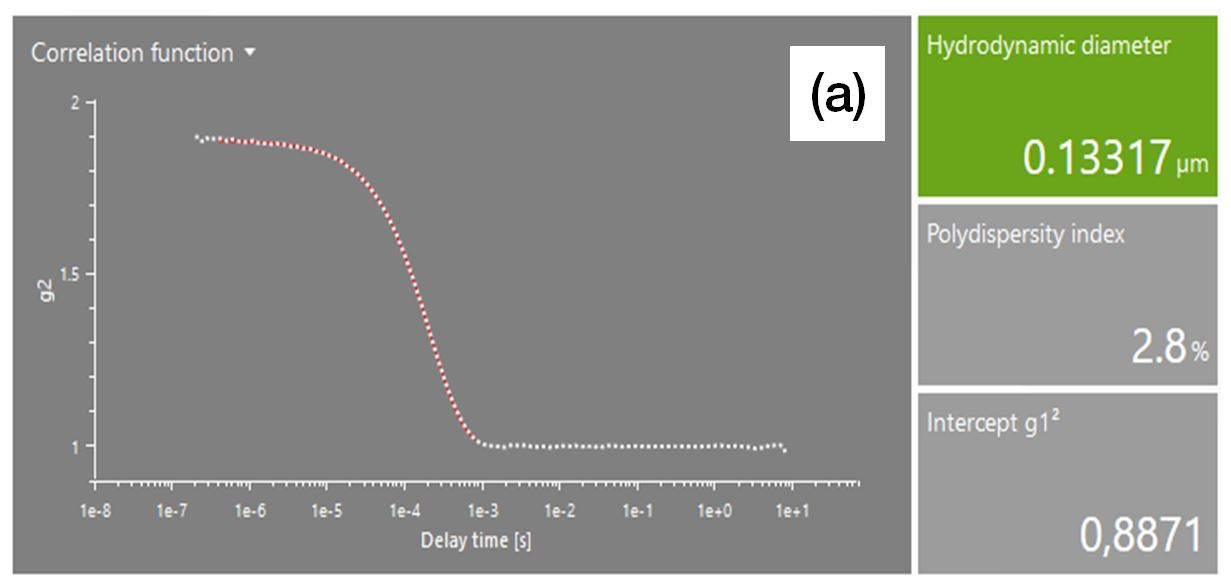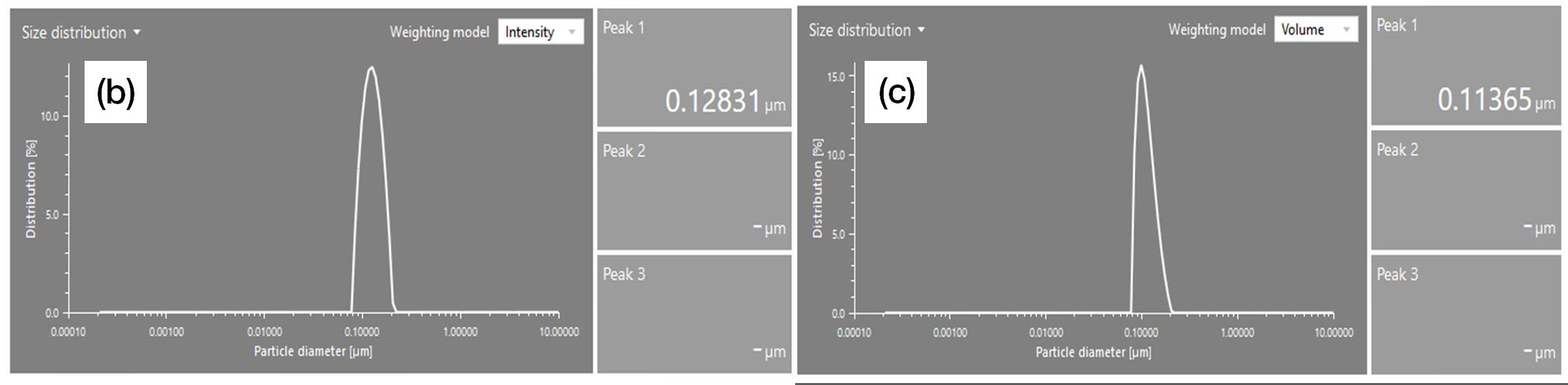0 Rates

# Particle size analysis methods: Dynamic light scattering vs. laser diffraction

There are two well-established methods for determining the particle size of a sample: dynamic light scattering and laser diffraction. Choosing which particle size analysis method to use requires consideration of the expected size range, the sample type (liquid or solid), the amount of available sample, the chemical stability, and the application field. However, the physical principle behind each technique is also important and the choice of the most suitable one is not always straightforward.
This article describes the principal differences between dynamic light scattering and laser diffraction and also provides an overview of the options for data interpretation and possible comparison.

## Comparison of particle sizing methods: laser diffraction vs. dynamic light scattering

Characteristics Dynamic light scattering (DLS) Laser diffraction (LD)
Assumption
Particles are spherical
Size range 0.3 nm to 10 µm 40 nm to 2500 µm
Theory of particle size measurement
• The velocity of particle movement is a function of the particle size.
• The movement of dispersed particles causes intensity fluctuations of scattered light which are measured and converted to particle size and particle size distribution (Stokes-Einstein equation).
- The diffracted/scattered intensity vs angle (diffraction pattern) is a function of the particle size.
- The final particle size distribution is obtained from the sum of diffraction patterns produced by the particles randomly oriented along the direction of the laser beam.
Equivalent diameter*

Hydrodynamic diameter (HDD):

diameter of a sphere with the same translational diffusion coefficient D as the particle in the same fluid under the same conditions, defined via the Stokes-Einstein equation:where kB is the Boltzmann constant, T the absolute temperature, and η the viscosity of the liquid medium, D is the translational diffusion coefficient

Laser diffraction equivalent diameter:
diameter of a sphere having on the cross-sectional area the same diffraction pattern as the investigated particle (1).
For particles ≤0.1 µm, the definition can be extended into volume-equivalent diameter.
In this case, the cross-sectional area becomes nearly the same as that of a sphere with equal volume (2).
Initial weighting model Intensity-based
• intensity fluctuations of scattered light
Volume-based
• the particle’s contribution relates to its volume equivalent to mass for constant density
Mean particle size result Monodisperse sample:

Hydrodynamic mean diameter (HDD)
Polydisperse sample: Peak analysis of particle size distribution graph
• D[4,3] , mean volume diameter
(De Brouckere Mean Diameter)
Parameters of the particle population
• ISO-defined polydispersity index (PDI)
• Peak analysis (area under the curve, standard deviation)
• D-values (e.g. D10, D50, D90)
• D-values (e.g. D10, D50, D90)
Other weighting models Recalculation of the intensity-based distribution to a number- and volume-based one is possible when the refractive index of the particles is known. Recalculation of the volume-based distribution to a surface- and number-based one is possible. The refractive index does not have any function in this case.
ISO norm ISO 22412 ISO 13320

*According to the IUPAC definition, the equivalent diameter of a non-spherical particle is equal to a diameter of a spherical particle that exhibits identical properties (e.g. aerodynamic, hydrodynamic, optical, electrical) to that of the investigated non-spherical particle.

## Results and data interpretation

Is it possible to compare DLS results with laser diffraction results? Which parameters should be compared?
The DLS particle size analysis method and laser diffraction technique provide results which are based on different equivalent diameters and weighting models.

For this reason, for many samples results measured with DLS and laser diffraction are not directly comparable and a deviation of 10 % or more between the results is to be expected. To decrease the deviation, the same weighting model should be used for comparison. When using the dynamic light scattering technique it is possible to recalculate the volume-weighted distribution as well as D-values.

However, any kind of recalculation will introduce an error which adds to the initial one related to the assumption of spherical particles. Therefore, calculations from the raw data are always more reliable than a recalculation.

Understanding the difference between DLS and laser diffraction results

The following considerations are crucial for understanding the difference in the results and the inaccuracy of the comparison:

• In order to directly compare the technologies the results of a DLS measurement have to be recalculated to obtain a volume-based distribution.
• Mean size is based on different weightings: D[4,3] based on volume; HDD based on intensity.
• The median (D50) should be considered to get a more accurate “average particle size”.

### Dynamic light scattering (DLS) results

DLS measurements provide results as the hydrodynamic diameter (HDD) and peak size (see Figure 1, 2).Figure 1: Exemplary correlation function of a DLS measurement. From there the HDD and the polydispersity index (PDI) are calculated.

The HDD cannot be measured by laser diffraction while the median of the peak size may be obtained by using further calculations. Therefore, the recalculation of the particle size distribution from intensity- to volume-based and then the calculation of the volume-weighted D-values are necessary for a reasonable comparison.

By means of the material refractive index the intensity-based result can be recalculated into the volume-based one.Figure 2: Particle size and size distribution of polystyrene latex measured by DLS: (a) correlation function and hydrodynamic diameter (HDD); (b) and (c) particle size distribution and peak size in the intensity- and volume-based distribution, respectively

### Laser diffraction (LD) results

The D-values provided by laser diffraction results do not give information about the peak size but describe the whole particle size distribution (see Figure 3). In order to define the particle size of the investigated sample, the median or the mean size (e.g. D [4,3]) has to be considered.

### Comparison of DLS and LD results

It can be observed that although there is a certain similarity (difference smaller than 10 %) between the HDD intensity-weighted (DLS: 0.13 µm) and the D50 volume-weighted (LD: 0.14 µm), the comparison would not be accurate because they have two different meanings (D50 is not a mean but a median) and they are based on different weighting models.

For this reason, from the recalculated DLS volume-based distribution, the D-values can be determined and used for the comparison with the laser diffraction results (see Table 1). The comparison between the volume-weighted D-values is shown below. The difference is higher than 20 %.

Instrument D10 [µm] D50 [µm] D90 [µm]
LD 0.06 0.15 0.34
DLS 0.08 0.10 0.14

Table 1: Comparison of volume-weighted D-values derived from DLS and LD for the polystyrene latex

The difference in results obtained from the two different techniques might be confusing at first glance, thus making the decision of which technique to choose even more challenging. In this case, however, it is useful to consider the sample type.

## Which technique for which application?

The following points should be taken into account:

Conditions DLS LD
Sample type Liquid dispersions (liquid/liquid, powder/liquid) Liquid dispersions, dry powders
Sample amount available Liquid sample: µL to mLDry samples: mg to g Liquid sample: mLDry samples: g
Sample recovery Always possible
Temperature control Always possible, typically from 0 °C up to 90 °C Possible to measure at different temperatures with the suitable accessory
Quality parameters
• low relative standard deviation (RSD, %; e.g. <5 %) over a repetition series
• detector signal (intensity trace)
• intercept and baseline of the correlation functionpeak analysis
• low relative standard deviation (RSD, %; e.g. <5 %) for D-values over a repetition series
• fit error
• detector signals
Method development No method optimization necessary Method optimization necessary for new samples
Measurements of highly concentrated liquid samples Possibility of measuring undiluted samples up to 70 % (m/v), otherwise pre-dilution is possible before filling the cuvette The sample is directly diluted in the liquid dispersion unit. The stirrer, ultrasound, and the circulation help to adjust the particle concentration (obscuration) in the instrument.
Lower size limit Primary particles in the lower nanometer range (typical lower size range 0.3 nm) LD is less sensitive to the weak signals coming from small particles in the nanometer range (<100 nm) and the accuracy can be limited.
Measurement time Both techniques are very fast (a few minutes). In some cases, laser diffraction measurements can be faster than DLS measurements.
Detection of impurities Small contaminants in a very low concentration will be observed by dynamic light scattering more readily than by laser diffraction.
Dispersion stability Laser diffraction is more suitable for analyzing samples that have large particles (>10 µm). The intensity signal is stronger and results are related to the particle volume; dispersion via ultrasound, stirring, and circulation ensure that particles remain in suspension. There is no sedimentation risk like with the DLS technique.
Statistics The accuracy of results and the repeatability (relative standard deviation %) have to be considered, e.g. RSD <5 %.
Typical applications
• Research and quality control:
• biosciences (e.g. proteins, antibodies)
• pharmaceuticals (e.g. liposomes, emulsions)nanoparticles (e.g. gold nanoparticles)
• Quality control:
• building materials (e.g. cement, concrete, sand)
• petrochemical (e.g. drilling fluid)pharmaceutical powders (e.g. API, excipients)

## Conclusion

Laser diffraction and dynamic light scattering are particle sizing methods which are well-established in both research and industry. Deciding on which technique to use for the particle analysis of a specific substance requires consideration of a number of factors, including the goal of the measurement (e.g. measurement of primary particles or agglomerates), the available sample amount, ease of sample preparation, measurement duration, and the nature of expected impurities. Results measured by laser diffraction can be compared with results measured by DLS and vice versa but this comparison must always be assumed to include a significant deviation. Therefore, comparison is best carried on results determined using the same technique.

Learn more about dynamic light scattering which is used in particle analyzers such as the Litesizer series